# Toxboot: A Field Guide

#### 2016-08-23

Toxboot is a package designed to quantify uncertainty in concentration response curves observed in high throughput screening. Using bootstrap resampling, the uncertainty in fitting model curves to the experimental data is determined. This is achieved by resampling with replacement from the experimental concentration response values, and adding to the resampled values normally distributed noise equivalent to the noise in the baseline response. The current implementation is designed to be used with USEPA’s ToxCast data and the tcpl package using tcpl::tcplFit so that the same fitting routine is used for the point estimates and bootstrap resample fitting. The workflow can be broken into three steps:

• Get the data into the correct format
• Bootstrap the data, fit each bootstrap sample, and write the results to a database, memory, or file
• Read the results from the database, memory, or file and perform calculations or plot the results

This workflow is described in detail below.

library(tcpl)
library(toxboot)
library(data.table)
library(RMySQL)
library(DBI)
library(magrittr)
library(ggplot2)
library(pander)

## Input Data Format

Once a working version of the tcpl database and package is setup, data preparation for bootstrap resampling is handled by the function toxbootQueryToxCast. Simply pass this function a vector assay endpoint id (aeid) values and it will query the toxcast database to get all of the concentration response values for the aeids, merge the corresponding parameter fid id (m4id) values, and calculate a basedline median absolution deviation (bmad). The data will then be in a format ready for bootstrapping. An included dataset erl3data used in examples throughout this vignette was generated from the October 2015 tcpl database prod_external_invitrodb_v2 using toxbootQueryToxCast for a select group of aeids and m4ids using the following code. A subset of m4ids was selected in this case to reduce the datasize included with the package.

tcplConf(db = "prod_external_invitrodb_v2")
assay_names <- c("NVS_NR_bER",
"OT_ER_ERaERa_1440",
"ATG_ERa_TRANS_up",
"TOX21_ERa_LUC_BG1_Agonist",
"ACEA_T47D_80hr_Positive")

aeid_table <- aeid_table_full[aenm %in% assay_names]
aeids <- aeid_table[,aeid]

dat <- toxbootQueryToxCast(aeids = aeids)

set.seed(12345)
m4ids <- sample(unique(dat[, m4id]), size = 200)
erl3data <- dat[m4id %in% m4ids]

## Bootstrap

Once the data is formatted correctly using toxbootQueryToxCast it is ready to be bootstrapped. The function toxbootmc is the highest level function to calculate the bootstrapping, and is explored in the subsections below. Under the hood, this function does some filtering of m4ids if writting to mongoDB and then runs the function toxboot using mclapply so that the bootstrapping will be multicore. Note that the parameter cores which will be passed as mc.cores to the mclapply function, specifies the number of cores to use and must be set to 1 if running in a windows environment.

The steps within toxboot are:

• The toxboot function calls toxbootReplicates to perform the the sampling.
• A matrix of sampled responses is returned.
• toxboot then applies the function tcpl::tcplFit to every sample.
• Each sample fitting result is stored in a data table.
• Finally, the results are written to the user specified location.

Options for the destination of results are memory (default), file, mongo, or mysql, as explored in the following subsections.

### Memory

dat <- toxbootmc(dat = erl3data,
boot_method = "smooth",
m4ids = tail(erl5data[hitc == 1L, m4id], 10),
cores = 1,
destination = "memory",
replicates = 10)
dim(dat)
##  100  52

### File

toxbootmc(dat = erl3data,
boot_method = "smooth",
cores = 8,
destination = "file",
replicates = 10)

### MongoDB

Connection parameters for the mongoDB are set using toxbootConf().

toxbootConf(mongo_host = "123.45.67.89",
db = "bootstrap",
DBNS = "bootstrap.prod_external_invitrodb_v2",

toxbootmc(dat = erl3data,
boot_method = "smooth",
cores = 8,
destination = "mongo",
replicates = 10)

Read the results back using toxbootGetMongoFields. The parameters passed to the function will form the basis of the query. The parameter fields will specify which values to return. In the example below, the function query any documents which have an m4id found in the erl3data dataset and will return the hill and gnls model parameters, the aic values for the 3 models, and the max_med for these documents. These parameters are the minimum necessary to calculate the winning model and determine the activity call. In addition, the parameter m4id is returned to be used as an index in the resulting data.table.

m4ids <- unique(erl3data[, m4id])
fields <- c("m4id", "max_med", "hill_ga", "hill_gw", "hill_tp", "hill_aic",
"gnls_ga", "gnls_gw", "gnls_tp",  "gnls_la", "gnls_lw", "gnls_aic",
"cnst_aic")
dat_boot <- toxbootGetMongoFields(m4id = m4ids, fields = fields)

### MySQL

With destination = "mysql" the bootstrap results will be written to a table toxboot.

Authentication and connection parameters are handled using a MySQL configuration file as recommended by the RMySQL package. This file can be used to maintain all of your MySQL parameters, which can then be accessed by name.

[client]
host = website.com

[toxboot]
database = dev_toxboot

If the table toxboot has not already been setup or has been setup incorrectly, the following command will drop the table and start over from scratch

toxbootMysqlCreateTable()
toxbootmc(dat = erl3data,
boot_method = "smooth",
cores = 32,
destination = "mysql",
replicates = 10)

dat_boot <- toxbootGetMySQLFields()

## Analyzing results

### Model Selection and Hit Call

The toxboot and toxbootmc functions perform the equivalent of the mc4 calculation in package tcpl. This calculation uses tcpl::tcplFit to use Level 3 (concentration response) data to calculate Level 4 (model fit parameter) values. Often, we are interested in looking at the winning model and the hit call. The function toxbootHitParamCI performs the same logic as Level 5 (model selection and hit call) calculation in tcpl, choosing a winning model and making a hit call. This function needs to know the cutoff to use in making the hit call. The easiest way to accomplish this is to pass the function a Level 5 dataset for every assay. An example of a Level 5 dataset corresponding to the example erl3data dataset was generated using the command:

m4ids <- unique(erl3data[, m4id])
erl5data <- tcplLoadData(5, fld = "m4id", val = m4ids, type = "mc")

Using the result, dat, from the Memory example above, calculate the winning model and hit call for each bootstrap resample using:

dat_tb <- toxbootHitParamCI(dat, erl5data)

#### Hit Percents

One benefit of resampling is the activity determination, or hit call, can be given a percent or probability rather than a binary designation. To calculate this on the results from using toxbootHitParamCI:

dat_sum <- dat_tb[, .(hit_pct = sum(boot_hitc)/10), by = m4id]
dat_sum
##        m4id hit_pct
##  1: 8507493     1.0
##  2: 8507963     0.6
##  3: 9056786     1.0
##  4: 9056819     1.0
##  5: 9056965     0.9
##  6: 9057063     1.0
##  7: 9057334     1.0
##  8: 9057437     1.0
##  9: 9057585     0.4
## 10: 9057756     1.0
ggplot(dat_sum,
aes(x = hit_pct)) +
geom_histogram(binwidth = 0.1) +
theme_bw()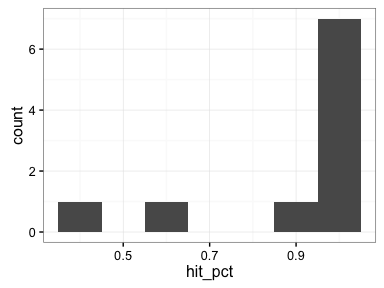Use an ecdf plot to visualize, removing the impact of the choice of binwidth.

ggplot(dat_sum,
aes(x = hit_pct)) +
stat_ecdf() +
theme_bw()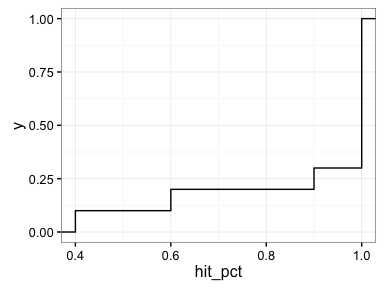#### Model Parameters

Let’s take a look at a specific curve, m4id = 9057756. The point estimates from the ToxCast database are:

pander(erl5data[m4id == 9057756, .(modl,
hill_ga,
hill_gw,
hill_tp,
gnls_ga,
gnls_gw,
gnls_tp,
gnls_la,
gnls_lw)],
split.table = Inf)
modl hill_ga hill_gw hill_tp gnls_ga gnls_gw gnls_tp gnls_la gnls_lw
hill -0.1952 1.991 93.46 -0.1952 1.991 93.46 3.497 17.97

As you can see, the gnls gain parameters are the same as the hill parameters. Plotting the points and the gnls and hill curves shows the fit curve relative to the data.

  hill_ga <- erl5data[m4id == 9057756, hill_ga]
hill_gw <- erl5data[m4id == 9057756, hill_gw]
hill_tp <- erl5data[m4id == 9057756, hill_tp]
gnls_ga <- erl5data[m4id == 9057756, gnls_ga]
gnls_gw <- erl5data[m4id == 9057756, gnls_gw]
gnls_tp <- erl5data[m4id == 9057756, gnls_tp]
gnls_la <- erl5data[m4id == 9057756, gnls_la]
gnls_lw <- erl5data[m4id == 9057756, gnls_lw]

ggplot(erl3data[m4id == 9057756],
aes(x=logc,
y=resp)) +
stat_function(fun = hill_curve,
args=list(hill_tp = hill_tp,
hill_ga = hill_ga,
hill_gw = hill_gw),
alpha = 1,
color = "red",
size = 1) +
stat_function(fun = gnls_curve,
args=list(top = gnls_tp,
ga = gnls_ga,
gw = gnls_gw,
la = gnls_la,
lw = gnls_lw),
alpha = 1,
color = "blue",
size = 1,
linetype = 2) +
theme_bw() +
geom_point(size=5,alpha=1) +
theme(legend.position="none", legend.title=element_blank()) +
ylab("Percent Activity") +
xlab("Log Concentration")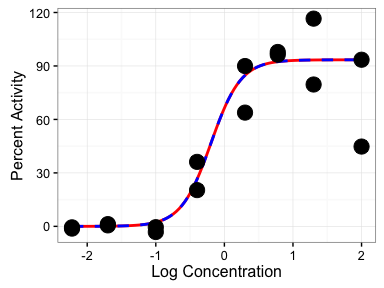The blue dashed line is the gnls model which fits exactly over the solid red line corresponding to the hill model.

We can then look at the results from bootstrap resampling to understand the variability in the modl_ga parameter. Using the dat_tb dataset calculated above, which had used 10 bootstap resamples:

ggplot(dat_tb[m4id == 9057756],
aes(x = modl_ga)) +
stat_ecdf() +
theme_minimal()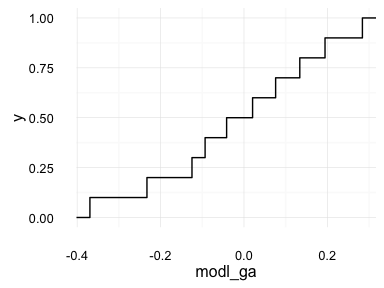Clearly 10 bootstrap replicates is not enough to get a good distribution. Let’s run 1,000 and 10,000 for m4id == 9057756 and see how this improves. Note the change in toxbootmc, where m4ids is now specified as rep(9057756, 8). When evaluated this gives a vector which allows the single curve to be bootstrapped on multiple processors. With replicates set to 125, this will give 8 x 125 = 1000 bootstrap resamples.

dat1000 <- toxbootmc(dat = erl3data,
m4ids = rep(9057756, 8),
boot_method = "smooth",
cores = 8,
destination = "memory",
replicates = 125) %>%
toxbootHitParamCI(erl5data)

dat10000 <- toxbootmc(dat = erl3data,
m4ids = rep(9057756, 8),
boot_method = "smooth",
cores = 8,
destination = "memory",
replicates = 1250) %>%
toxbootHitParamCI(erl5data)

The data from the above calculation is included with the package and can be loaded directly

dim(dat1000)
##  1000   59
dim(dat10000)
##  10000    59

The results from 10, 1000, and 10000 resamples can be compared.

ggplot(dat10000,
aes(x = modl_ga)) +
stat_ecdf() +
stat_ecdf(data = dat1000,
color = "blue",
linetype = 2) +
stat_ecdf(data = dat_tb[m4id == 9057756],
color = "red",
linetype = "dotdash") +
theme_bw()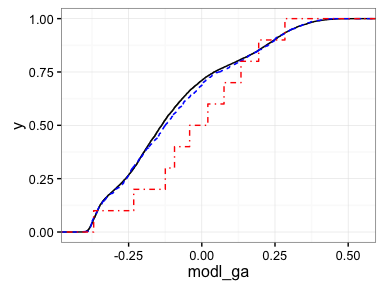Both the solid black line corresponding to 10,000 replicates and the dashed blue line for 1,000 replicates are clearly smoother than the results from only 10 resamples (red dotdash line). The difference between 1,000 and 10,000 is much less significant. Comparisons like this plot allow the optimimum number of bootstrap resamples to be selected. In this case, since 1,000 and 10,000 have similar distributions, there is no need to calculate 10,000 replicates if the goal is to calculate a 95% confidence interval. Choosing 10 replicates, however, would lead to a different result even at a 50% confidence interval.

Next, we can plot the fit curves to visualize the uncertainty in the fitting.

rep_num <- 1000
xmin <- min(erl3data[m4id == 9057756, logc])
xmax <- max(erl3data[m4id == 9057756, logc])

dat_boot_curve <- expand.grid(replicate = 1:rep_num,
lconc = seq(xmin,
xmax,
length.out = 100)) %>%
data.table()
dat_result <- copy(dat1000)
dat_result[, repnum := 1:.N]
dat_boot_curve <- merge(dat_boot_curve,
dat_result,
by.x = "replicate",
by.y = "repnum")
dat_boot_curve[modl == "hill",
resp := hill_curve(hill_tp = hill_tp,
hill_ga = hill_ga,
hill_gw = hill_gw,
lconc)]
dat_boot_curve[modl == "gnls",
resp := gnls_curve(top = gnls_tp,
ga = gnls_ga,
gw = gnls_gw,
la = gnls_la,
lw = gnls_lw,
lconc)]

hill_ga <- erl5data[m4id == 9057756, hill_ga]
hill_gw <- erl5data[m4id == 9057756, hill_gw]
hill_tp <- erl5data[m4id == 9057756, hill_tp]
gnls_ga <- erl5data[m4id == 9057756, gnls_ga]
gnls_gw <- erl5data[m4id == 9057756, gnls_gw]
gnls_tp <- erl5data[m4id == 9057756, gnls_tp]
gnls_la <- erl5data[m4id == 9057756, gnls_la]
gnls_lw <- erl5data[m4id == 9057756, gnls_lw]

ggplot(dat_boot_curve,
aes(x=lconc,
y=resp,
color = modl)) +
geom_line(size = 2,
alpha = 0.01,
aes(group = replicate)) +
geom_point(data = erl3data[m4id == 9057756],
aes(x = logc,
y = resp),
alpha = 1,
size = 5,
color = 'black',
fill = 'cyan',
shape=21) +
stat_function(fun = hill_curve,
args=list(hill_tp = hill_tp,
hill_ga = hill_ga,
hill_gw = hill_gw),
alpha = 1,
color = "cyan",
size = 1) +
scale_color_manual(values = c("hill" = "red", "gnls" = "blue")) +
ylab("Percent Activity") +
xlab("Log Concentration (uM)") +
expand_limits(y = c(120, -40)) +
theme_bw() +
guides(color=FALSE)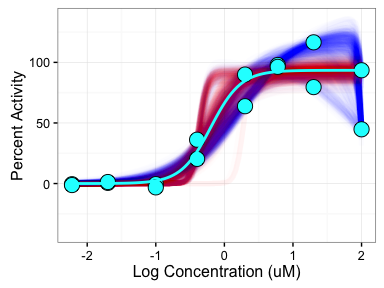In this plot, the cyan circles are the experimental concentration response values, the cyan curve is the point estimate winning model from the ToxCast database, and the red and blue curves correspond to the 1000 bootstrap resample winning models colored by the winning model (hill = red, gnls = blue). For this dataset, the winning model varies between the hill and gnls depending on the exact points resampled, showing the uncertainty in the model selection process.